0
90

Practice IIFT solved Syllogisms Questions paper tests, which are the practice question to have a firm grasp on the Syllogisms topic in the IIFT exam. In this article, one can find the top 20 very Important Syllogisms Questions for IIFT based on asked questions in previous exam papers. The IIFT question papers contain actual questions asked with detailed solutions. Download these important Syllogisms Questions for IIFT PDF with detailed solutions. Click on the link given below to download the PDF.

Question 1: Two statements are given, followed by three conclusions numbered I, II and III.
Assuming the statements to be true, even if they seem to be at variance with
commonly known facts, decide which of the conclusions logically follow(s) from the statements.
Statements:
All Birds are Sparrows.
All Sparrows are Reptiles.
Conclusions:
I. Some Reptiles are Birds.
II. All Reptiles are Sparrows.
III. All Birds are Reptiles.

a) Both conclusions II and III follow.

b) Both conclusions I and II follow.

c) Neither conclusion I, nor II, and III follow.

d) Both conclusions I and III follow.

Solution: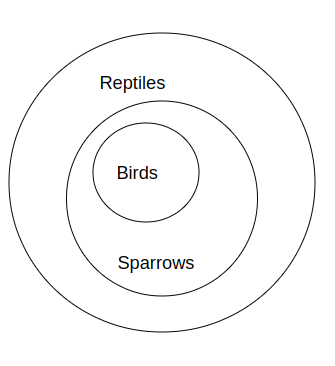As per the Statements given in question, we get the above Venn diagram,

Conclusion :

1.  Some reptiles are birds. : True (clearly seen all birds are reptiles so, it follows.)

2.All Reptiles are Sparrows : False (All sparrows are reptiles but its vice-versa is not possible.)

3. All birds are reptiles : True (All birds are sparrows and all sparrows are reptiles.,so all birds are reptiles.)

Hence, Conclusion 1 and 3 follows,

Hence, Option D is correct.

Question 2: Read the given statements and conclusions carefully. Assuming that the information given in the statements is true, even if it appears to be at variance with commonly known facts, decide which of the given conclusions logically follows from the statements.
Statements:
1. All malls are kings.
2. No king is a heater.
Conclusions:
I. No mall is a heater.
II. All kings are malls.

a) Either conclusion I or II follows.

b) Only conclusion II follows.

c) Only conclusion I follows.

d) Neither conclusion I nor II follows.

Solution:

The basic diagram for the given statements is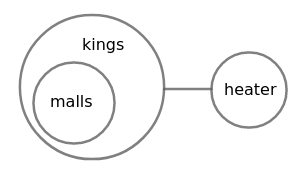I. No mall is a heater

From the basic diagram, no mall is a heater. Hence conclusion I follows.

II. All kings are malls

From the basic diagram, all kings are not malls. Hence conclusion II do not follow.

$\therefore\$Only conclusion I follows the given statements.

Hence, the correct answer is Option C

Question 3: Read the given statements and conclusions carefully. Assuming that the information given in the statements is true, even if it appears to be at variance with commonly known facts, decide which of the given conclusions logically follow(s) from the statements.
Statements:
No plastic is iron.
All steel is iron.
Conclusions:
I. Some plastic is steel.
II. Some iron is steel.
III. No steel is plastic.

a) Only conclusions I and III follow.

b) Only conclusions II and III follow.

c) Only conclusions I and II follow.

d) Only conclusion II follows.

Solution:

The basic diagram for the given statements is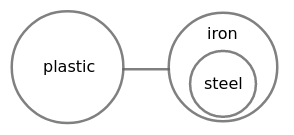I. Some plastic is steel

From the basic diagram, no plastic is steel. Hence conclusion I do not follow.

II. Some iron is steel

From the basic diagram, some iron is steel. Hence conclusion II follows.

III. No steel is plastic

From the basic diagram, no steel is plastic. Hence conclusion III follows.

$\therefore\$Only conclusions II and III follow the given statements.

Hence, the correct answer is Option B

Question 4: Read the given statements and conclusions carefully. Assuming that the information given in the statements is true, even if it appears to be at variance with commonly known facts, decide which of the given conclusions logically follow(s) from the statements.
Statements:
1. Most teachers are women.
2. Some women are managers.
Conclusions:
I. Some managers are women.
II. Some teachers are managers.

a) Both conclusions I and II follow.

b) Only conclusion I follows.

c) Neither conclusion I nor II follows.

d) Only conclusion II follows.

Solution:

The basic diagram for the given statements isI. Some managers are women

From the basic diagram, some managers are women. Hence conclusion I follows.

II. Some teachers are managers

From the basic diagram, no teacher is manager. Hence conclusion II do not follow.

$\therefore\$Only conclusion I follows the given statements.

Hence, the correct answer is Option B

Question 5: Read the given statements and conclusions carefully. Assuming that the information given in the statements is true, even if it appears to be at variance with commonly known facts, decide which of the given conclusions logically follow(s) from the statements.
Statements:
1. Some trees are silver oaks.
2. Some silver oaks are tall objects.
Conclusions:
I. Some tall objects are silver oaks.
II. All tall objects are trees.

a) Only conclusion I follows.

b) Only conclusion II follows.

c) Neither conclusion I nor II follows.

d) Both conclusions I and II follow.

Solution:

The basic diagram for the given statements is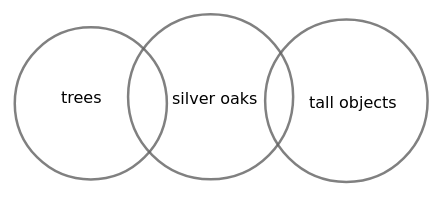I. Some tall objects are silver oaks

From the basic diagram, some tall objects are silver oaks. Hence conclusion I follows.

II. All tall objects are trees

From the basic diagram, no tall object is tree. Hence conclusion II do not follow.

$\therefore\$Only conclusion I follows the given statements

Hence, the correct answer is Option A

Question 6: Read the given statements and conclusions carefully. Assuming that the information given in the statements is true, even if it appears to be at variance with commonly known facts, decide which of the given conclusions logically follow(s) from the statements.
Statements:
All speakers are equipment.
All equipment are wires.
Conclusions:
I. Some wires are equipment.
II. No speaker is wire.
III. Some speakers are not wires.

a) Both conclusion I and III follow.

b) Only conclusion I follows.

c) All conclusions I, II and III follow.

d) Only conclusion III follows.

Solution:

The basic diagram for the given statements is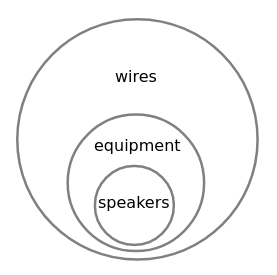I. Some wires are equipment

From the basic diagram, some wires are equipment. Hence conclusion I follows.

II. No speaker is wire

From the basic diagram, all speakers are wires. Hence conclusion II do not follow.

III. Some speakers are not wires

From the basic diagram, all speakers are wires. Hence conclusion III do not follow.

$\therefore\$Only conclusion I follows the given statements.

Hence, the correct answer is Option B

Question 7: Read the given statements and conclusions carefully. Assuming that the information given in the statements is true, even if it appears to be at variance with commonly known facts, decide which of the given conclusions logically follow(s) from the statements.
Statements:
1. No cup is a book.
2. Apple is a cup.
Conclusions:
I. Apple is not a book.
II. Apple may or may not be a book.

a) Both conlusions I and II follow.

b) Only conclusion II follows.

c) Neither conclusion I nor II follows.

d) Only conclusion I follows.

Solution:

The basic diagram for the given statements is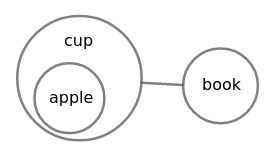I. Apple is not a book

From the basic diagram, apple is not book. Hence conclusion I follows.

II. Apple may or may not be a book

From the basic diagram, apple is not book definitely. Hence conclusion II do not follow.

$\therefore\$Only conclusion I follows the given statements.

Hence, the correct answer is Option D

Question 8: Read the given statements and conclusions carefully. Assuming that the information given in the statements is true, even if it appears to be at variance with commonly known facts, decide which of the given conclusions logically follow(s) from the statements.
Statements:
1. All doors are teachers.
2. All teachers are cups.
Conclusions:
I. All cups are doors.
II. All doors are cups.
III. All teachers are doors.
IV. Some cups are teachers.

a) Only conclusions I and II follow.

b) Only conclusion I follows.

c) Only conclusions II and IV follow.

d) Only conclusions I and III follow.

Solution:

The basic diagram for the given statements is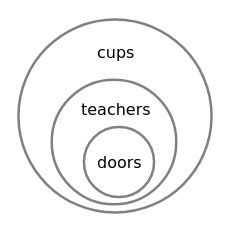I. All cups are doors

From the basic diagram, all cups are not doors. Hence conclusion I do not follow.

II. All doors are cups

From the basic diagram, all doors are cups. Hence conclusion II follows.

III. All teachers are doors

From the basic diagram, all teachers are not doors. Hence conclusion III do not follow.

IV. Some cups are teachers

From the basic diagram, some cups are teachers. Hence conclusion IV follows.

$\therefore\$Only conclusions II and IV follow the given statements

Hence, the correct answer is Option C

Question 9: Read the given statements and conclusions carefully. Assuming that the information given in the statements is true, even if it appears to be at variance with commonly known facts, decide which of the given conclusions logically follow(s) from the statements.
Statements:
1. Some cats are dogs.
2. Some trees are dogs.
Conclusions:
(I) Some dogs are cats.
(II) Some cats are trees.

a) Both conclusions I and II follow.

b) Either conclusion I or II follows.

c) Only conclusion II follows.

d) Only conclusion I follows.

Solution:

The basic diagram for the given statements is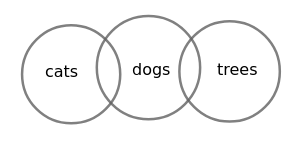(I) Some dogs are cats

From the basic diagram, some dogs are cats. Hence conclusion I follows.

(II) Some cats are trees

From the basic diagram, no cat is tree. Hence conclusion II do not follow.

$\therefore\$Only conclusion I follows the given statements.

Hence, the correct answer is Option D

Question 10: Read the given statements and conclusions carefully. Assuming that the information given in the statements is true, even if it appears to be at variance with commonly known facts, decide which of the given conclusions logically follow(s) from the statements.
Statements:
Some Pen are Ink.
Some Paper are Ink.

Conclusions:
(I) All Ink are Pen.
(II) Some Pens are Paper

a) Either conclusion I or conclusion II follows.

b) Only conclusion I follows.

c) Both conclusions I and II follow.

d) Neither conclusion I nor conclusion II follows.

Solution:

The basic diagram for the given figure is(I) All Ink are Pen

From the basic diagram, all ink are not pen. Hence conclusion I do not follow.

(II) Some Pens are Paper

From the basic diagram, no pen is paper. Hence conclusion II do not follow.

$\therefore\$Neither conclusion I nor conclusion II follows the given statements.

Hence, the correct answer is Option D

Question 11: Read the given statements and conclusions carefully. Assuming that the information given in the statements is true, even if it appears to be at variance with commonly known facts, decide which of the given conclusions logically follow(s) from the statements.
Statements:
All woods are roots.
All fruits are roots.
No woods are a fruit.

Conclusion:
(I) Some roots are fruits.
(II) All roots are woods.

a) Only conclusion II follows.

b) Neither conclusion I nor conclusion II follows

c) Only conclusion I follows.

d) Both conclusion I and II follows

Solution:

The basic diagram for the given statements is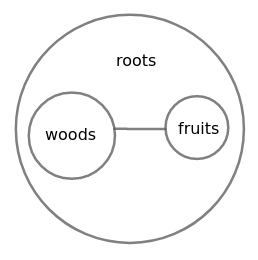(I) Some roots are fruits

From the basic diagram, some roots are fruits. Hence conclusion I follows.

(II) All roots are woods

From the basic diagram, all roots are not woods. Hence conclusion II do not follow.

$\therefore\$Only conclusion I follows the given statements

Hence, the correct answer is Option C

Question 12: Read the given statements and conclusions carefully. Assuming that the information given in the statements is true, even if it appears to be at variance with commonly known facts, decide which of the given conclusions logically follow(s) from the statements.
Statements:
1. Some bananas are green.
2. Some green are yellow.
Conclusions:
I. Some bananas are yellow.
II. Some yellow are bananas
III. All yellow are bananas.
IV. Some green are bananas.

a) Only conclusions I, II and IV follow.

b) Only conclusions II and IV follow.

c) Only conclusions I and IV follow.

d) Only conclusion IV follows.

Solution:

The basic diagram from the given statements is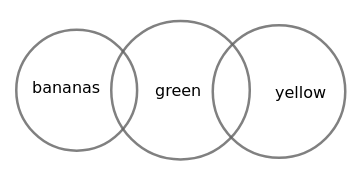I. Some bananas are yellow

From the basic diagram, some bananas are not yellow. Hence conclusion I do not follow.

II. Some yellow are bananas

From the basic diagram, some yellow are not bananas. Hence conclusion II do not follow.

III. All yellow are bananas

From the basic diagram, all yellow are not bananas. Hence conclusion III do not follow.

IV. Some green are bananas

From the basic diagram, some green are bananas. Hence conclusion IV follows .

$\therefore\$Only conclusion IV follows the given statements

Hence, the correct answer is Option D

Question 13: Read the given statements and conclusions carefully. Assuming that the information given in the statements is true, even if it appears to be at variance with commonly known facts, decide which of the given conclusions logically follow(s) from the statements.
Statements:
1. All squares are rectangles.
2. All rectangles are polygons.
Conclusions:
I. Square is not a polygon.
II. Square is both rectangle and polygon.

a) Only conclusion I follows.

b) Neither conclusion I nor II follows.

c) Only conclusion II follows.

d) Both conclusions I and II follow.

Solution:

The basic diagram for the given statements is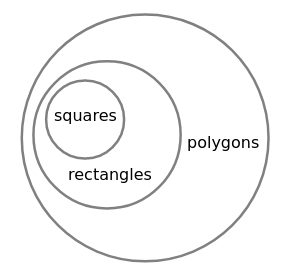I. Square is not a polygon

From the basic diagram, every square is a polygon. Hence conclusion I do not follow.

II. Square is both rectangle and polygon

From the basic diagram, every square is both rectangle and polygon. Hence conclusion II follows.

$\therefore\$Only conclusion II follows the given statements

Hence, the correct answer is Option C

Question 14: Read the given statements and conclusions carefully. Assuming that the information given in the statements is true, even if it appears to be at variance with commonly known facts, decide which of the given conclusions logically follow(s) from the statements.
Statements:
1. Some bananas are mangoes.
2. Some mangoes are cats.
Conclusions:
I. All cats are bananas.
II. Some cats are mangoes.

a) Only conclusion II follows.

b) Neither conclusion I nor II follows.

c) Both conclusions I and II follow.

d) Only conclusion I follows.

Solution:

The basic diagram for the given statements is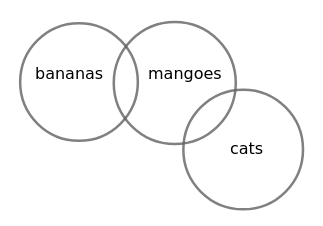I. All cats are bananas

From the basic diagram, all cats are not bananas. Hence conclusion I is not correct.

II. Some cats are mangoes

From the basic diagram, some cats are mangoes. Hence conclusion II is correct.

$\therefore\$Only conclusion II follows the given statements

Hence, the correct answer is Option A

Question 15: Select the Venn diagram that best illustrates the relationship between the following classes.

a)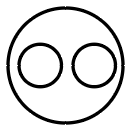b)c)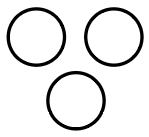d)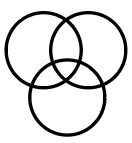Solution:

Fruits and Cookies are two different things where both of them are Eatables.

$\therefore\$The Venn diagram that best illustrates the relationship between the given classes is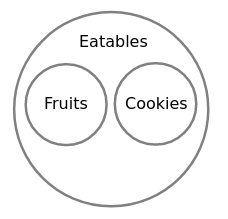Hence, the correct answer is Option A

Question 16: Read the given statements and conclusions carefully. Assuming that the information given in the statements is true, even if it appears to be at variance with commonly known facts, decide which of the given conclusions logically follow(s) from the statements.
Statements:
1. All cars are motors.
2. All motors are cycles.
Conclusions:
I. All cycles are cars.
II. All cars are cycles.

a) Only conclusion II follows

b) Neither conclusion I nor II follows.

c) Only conclusion I follows.

d) Both conclusions I and II follow.

Solution:

The basic diagram for the given statements is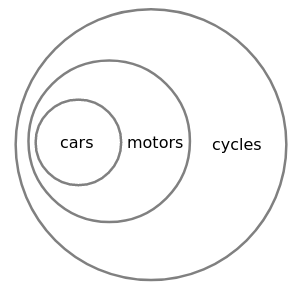I. All cycles are cars

From the basic diagram, all cycles are not cars. Hence conclusion I do not follow.

II. All cars are cycles

From the basic diagram, all cars are cycles. Hence conclusion follows.

$\therefore\$Only conclusion II follows the given statements

Hence, the correct answer is Option A

Question 17: Read the given statements and conclusions carefully. Assuming that the information given in the statements is true, even if it appears to be at variance with commonly known facts, decide which of the conclusions logically follow(s) from the statements.
Statements:

1. All bottles are jugs.
2. Some jugs are glasses.
Conclusions:

I. Some jugs are bottles.
II. Some glasses are jugs.
III. All glasses are bottles.

a) Only conclusions I follows.

b) Only conclusions II and III follow.

c) Only conclusions II follows.

d) Only conclusions I and II follow.

Solution:

The basic diagram for the given figure is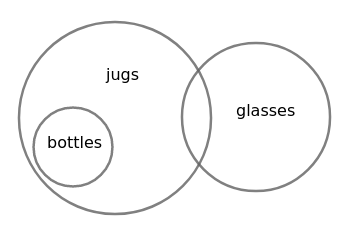I. Some jugs are bottles

From the basic diagram, some jugs are bottles. Hence conclusion I follows.

II. Some glasses are jugs

From the basic diagram, some glasses are jugs. Hence conclusion II follows.

III. All glasses are bottles

From the basic diagram, all glasses are not bottles. Hence conclusion III do not follow.

$\therefore\$Only conclusions I and II follow the given statements

Hence, the correct answer is Option D

Question 18: Read the given statements and conclusions carefully. Assuming that the information given in the statements is true, even if it appears to be at variance with commonly known facts, decide which of the given conclusions logically follow(s) from the statements.
Statements:

1. Some fishes are horses.
2. Some horses are kites.
Conclusions:

I. Some horses are fishes.
II. Some kites are fishes.
III. Some kites are horses.

a) Only conclusions II and IV follow.

b) Only conclusions I and II follow.

c) Only conclusions I and III follow.

d) All conclusions I, II and III follow.

Solution:

The basic diagram for the given statements is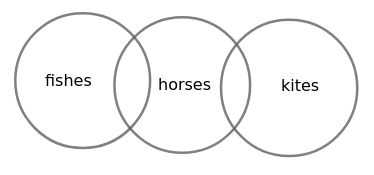I. Some horses are fishes

From the basic diagram, some horses are fishes. Hence conclusion I follows.

II. Some kites are fishes

From the basic diagram, no kite is fish. Hence conclusion II do not follow.

III. Some kites are horses

From the basic diagram, some kites are horses. Hence conclusion III follows.

$\therefore\$Only conclusions I and III follow the given statements

Hence, the correct answer is Option C

Question 19: Read the given statements and conclusions carefully. Assuming that the information given in the statements is true, even if it appears to be at variance with commonly known facts, decide which of the given conclusions logically follow(s) from the statements.
Statements:

I. Some books are tables.
II. All tables are chairs.
Conclusions:

I. All books are chairs.
II. Some books are chairs.
III. Some tables are book.
IV. All chairs are book.

a) Only conclusions I and IV follow.

b) Only conclusions II, III and IV follow.

c) Only conclusions II and III follow.

d) Only conclusion I follows.

Solution:

The basic diagram for the given statements is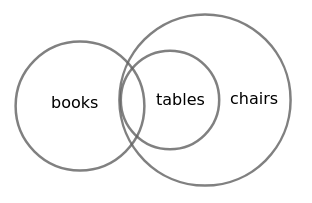I. All books are chairs

From the basic diagram, all books are not chairs. Hence conclusion I do not follow.

II. Some books are chairs

From the basic diagram, some books are chairs. Hence conclusion II follows.

III. Some tables are book

From the basic diagram, some tables are book. Hence conclusion III follows.

IV. All chairs are book

From the basic diagram, all chairs are not book. Hence conclusion IV do not follow.

$\therefore\$Only conclusions II and III follow the given statements

Hence, the correct answer is Option C

Question 20: Read the given statements and conclusions carefully. Assuming that the information given in the statements is true, even if it appears to be at variance with commonly known facts, decide which of the conclusions logically follow(s) from the statements.
Statements:

1. All boys are robots.
2. All girls are robots.
Conclusions:

I. Some robots are boys.
II. No robot is a girl.
III. Some robots are girls.

a) Only conclusion III follows.

b) Only conclusions I and II follow.

c) All conclusions I, II and III follow.

d) Only conclusions I and III follow.

Solution:

The basic diagram for the given statements is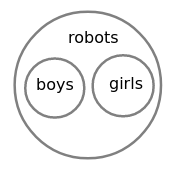I. Some robots are boys

From the basic diagram, some robots are boys. Hence conclusion I follows.

II. No robot is a girl

From the basic diagram, some robots are girls. Hence conclusion II do not follow.

III. Some robots are girls

From the basic diagram, some robots are girls. Hence conclusion III follows.

$\therefore\$Only conclusions I and III follow the given statements

Hence, the correct answer is Option D